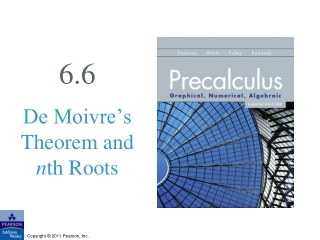DownloadDownload Presentation6.6

# 6.6

Download Presentation## 6.6

- - - - - - - - - - - - - - - - - - - - - - - - - - - E N D - - - - - - - - - - - - - - - - - - - - - - - - - - -
##### Presentation Transcript

1. 6.6 De Moivre’s Theorem and nth Roots

2. What you’ll learn about • The Complex Plane • Trigonometric Form of Complex Numbers • Multiplication and Division of Complex Numbers • Powers of Complex Numbers • Roots of Complex Numbers … and why The material extends your equation-solving technique to include equations of the form zn = c, n is an integer and c is a complex number.

3. Complex Plane

4. Absolute Value (Modulus) of a Complex Number

5. Graph of z = a + bi

6. Trigonometric Form of a Complex Number

7. Example Finding Trigonometric Form

8. Example Finding Trigonometric Form

9. Product and Quotient of Complex Numbers

10. Example Multiplying Complex Numbers

11. Example Multiplying Complex Numbers

12. A Geometric Interpretation of z2

13. De Moivre’s Theorem

14. Example Using De Moivre’s Theorem

15. Example Using De Moivre’s Theorem

16. Example Using De Moivre’s Theorem

17. nth Root of a Complex Number

18. Finding nth Roots of a Complex Number

19. Example Finding Cube Roots

20. Example Finding Cube Roots